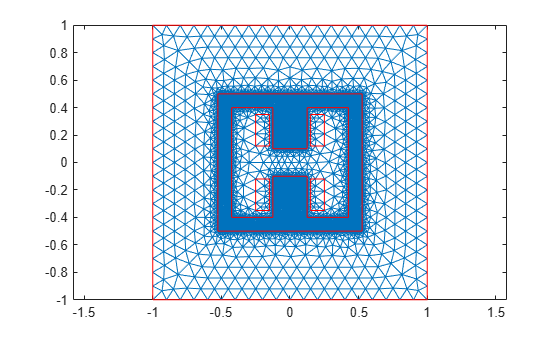# Magnetic Flux Density in H-Shaped Magnet

This example shows how to solve a 2-D magnetostatic model for a ferromagnetic frame with an H-shaped cavity. This setup generates a uniform magnetic field due to the presence of two coils.

Create a geometry that consists of a rectangular frame with an H-shaped cavity, four rectangles representing the two coils, and a unit square representing the air domain around the magnet. Specify all dimensions in millimeters, and use the value `convfactor = 1000` to convert the dimensions to meters.

`convfactor = 1000;`

Create the H-shaped geometry to model the cavity.

```xCoordsCavity = [-425 -125 -125 125 125 425 425 ... 125 125 -125 -125 -425]/convfactor; yCoordsCavity = [-400 -400 -100 -100 -400 -400 ... 400 400 100 100 400 400]/convfactor; RH = [2;12;xCoordsCavity';yCoordsCavity'];```

Create the geometry to model the rectangular ferromagnetic frame.

```RS = [3;4;[-525;525;525;-525;-500;-500;500;500]/convfactor]; zeroPad = zeros(numel(RH)-numel(RS),1); RS = [RS;zeroPad];```

Create the geometries to model the coils.

```RC1 = [3;4;[150;250;250;150;120;120;350;350]/convfactor; zeroPad]; RC2 = [3;4;[-150;-250;-250;-150;120;120;350;350]/convfactor; zeroPad]; RC3 = [3;4;[150;250;250;150;-120;-120;-350;-350]/convfactor; zeroPad]; RC4 = [3;4;[-150;-250;-250;-150;-120;-120;-350;-350]/convfactor; zeroPad];```

Create the geometry to model the air domain around the magnet.

```RD = [3;4;[-1000;1000;1000;-1000;-1000; ... -1000;1000;1000]/convfactor;zeroPad];```

Combine the shapes into one matrix.

`gd = [RS,RH,RC1,RC2,RC3,RC4,RD];`

Create a set formula and create the geometry.

```ns = char('RS','RH','RC1','RC2','RC3','RC4','RD'); g = decsg(gd,'(RS+RH+RC1+RC2+RC3+RC4)+RD',ns');```

Plot the geometry with the face labels.

```figure pdegplot(g,'FaceLabels','on')```Plot the geometry with the edge labels.

```figure pdegplot(g,'EdgeLabels','on')```Create a magnetostatic model and include the geometry in the model.

```model = createpde('electromagnetic','magnetostatic'); geometryFromEdges(model,g);```

Generate a mesh with fine refinement in the ferromagnetic frame.

```generateMesh(model,'Hface',{2,0.01},'Hmax',0.1,'Hgrad',2); figure pdemesh(model)```Specify the vacuum permeability value in the SI system of units.

`model.VacuumPermeability = 1.2566370614E-6;`

Specify a relative permeability of 1 for all domains.

`electromagneticProperties(model,'RelativePermeability',1);`

Now specify the large relative permeability of the ferromagnetic frame.

`electromagneticProperties(model,'RelativePermeability',10000,'Face',2);`

Specify the current density values on the upper and lower coils.

```electromagneticSource(model,'CurrentDensity',1E6,'Face',[5,6]); electromagneticSource(model,'CurrentDensity',-1E6,'Face',[4,7]);```

Specify that the magnetic potential on the outer surface of the air domain is 0.

`electromagneticBC(model,'Edge',[5,6,13,14],'MagneticPotential',0);`

Solve the model.

`R = solve(model)`
```R = MagnetostaticResults with properties: MagneticPotential: [26381x1 double] MagneticField: [1x1 FEStruct] MagneticFluxDensity: [1x1 FEStruct] Mesh: [1x1 FEMesh] ```

Plot the magnitude of the flux density.

```Bmag = sqrt(R.MagneticFluxDensity.Bx.^2 + ... R.MagneticFluxDensity.By.^2); pdeplot(model,'XYData',Bmag, ... 'FlowData',[R.MagneticFluxDensity.Bx ... R.MagneticFluxDensity.By])```### References

 Kozlowski, A., R. Rygal, and S. Zurek. "Large DC electromagnet for semi-industrial thermomagnetic processing of nanocrystalline ribbon." IEEE Transactions on Magnetics 50, issue 4 (April 2014): 1-4. https://ieeexplore.ieee.org/document/6798057.

## SupportGet trial now